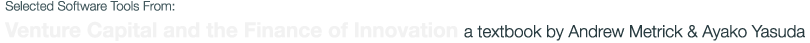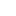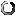# FLEX Calculator

The FLEX Calculator enables the simultaneous valuation of several random-expiration call options. The user inputs each component of an exit (or an LP) valuation equation, and the calculator values these components and adds them together.

## Assumptions

Total Valuation Volatility Interest Rate EHP

## Results

1- GP% LP value partial value

## Inputs

Delete Option Fraction Strike Type Call Value Fraction*Value
xloading application